### Home > MC2 > Chapter 3 > Lesson 3.2.3 > Problem3-87

3-87.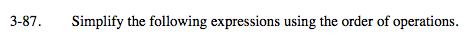Evaluate the terms in parentheses.
3(4) + 42 − (5)

Simplify the terms with exponents.
3(4) + 16 − (5)

Simpify the terms that are multiplied or divided.
12 + 16 − 5

Combine the terms that are added or subtracted.

23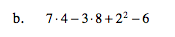Refer to the Math Notes box of Lesson 3.1.4.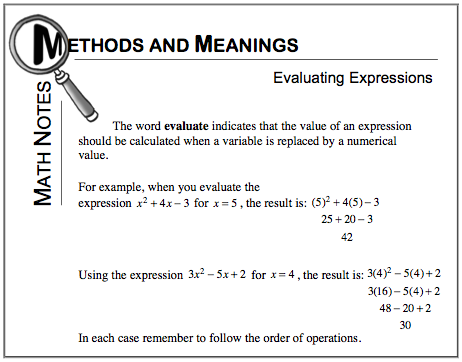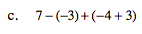See part (a) or (b).

9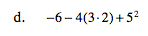See part (a) or (b).